# Bergman kernel function

Bergman kernel

A function of complex variables with the reproducing kernel property, defined for any domainin which there exist holomorphic functions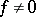of class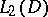with respect to the Lebesgue measure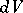. The function was introduced by S. Bergman . The set of these functionsforms the Hilbert space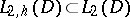with orthonormal basis;, whereis the space of holomorphic functions. The functionis called the Bergman kernel function (or simply the kernel function) of. The series on the right-hand side converges uniformly on compact subsets of, and belongs to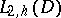for each given, the sum does not depend on the choice of the orthonormal basis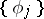. The Bergman kernel function depends on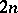complex variables, and is defined in the domain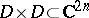; it has the symmetry property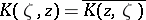, it is holomorphic with respect to the variableand anti-holomorphic with respect to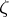. If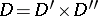,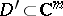,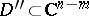, then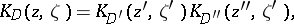where.

The most important characteristic of the Bergman kernel function is its reproducing property: For any function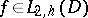and for any pointthe following integral representation is valid:Extremal properties of the Bergman kernel function are:

1) For any point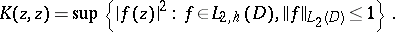2) Let a point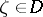be such that the classcontains functions satisfying the condition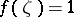. The functionthen satisfies this condition and has norm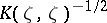, which is minimal for all such. The functionis called the extremal function of.

Changes in the Bergman kernel function under biholomorphic mappings are expressed in the following theorem: Ifis a biholomorphic mapping of a domainonto a domain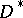,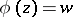,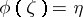, thenwhere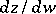is the Jacobian of the inverse mapping. Owing to this property the Hermitian quadratic form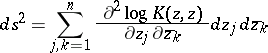is invariant under biholomorphic mappings.

The function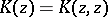, which is also called a kernel function, plays an important role in the intrinsic geometry of domains. In the general case it is non-negative, while the function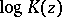is plurisubharmonic. In domainswhereis positive (e.g. in bounded domains), the functions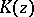andare strictly plurisubharmonic. The latter is tantamount to saying that in such domainsthe form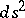is positive definite and, consequently, gives a Hermitian Riemannian metric in. This metric remains unchanged under biholomorphic mappings and is called the Bergman metric. It may be considered as a special case of a Kähler metric. It follows from extremal property 1) that the coefficients of the Bergman metric increase to infinity on approaching certain boundary points. If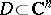is a strictly pseudo-convex domain or an analytic polyhedron, thenincreases to infinity for any approach ofto the boundary of the domain. Every domain which has this property of the Bergman kernel function is a domain of holomorphy.

For domains of the simplest type, the Bergman kernel function can be explicitly calculated. Thus, for the ball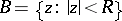in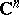, the Bergman function has the following form: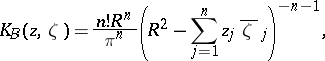and for the polydisc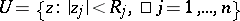, in: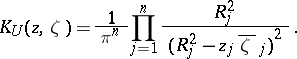In the special case whenandis the disc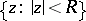in the complex-plane, the Bergman metric becomes the classical hyperbolic metric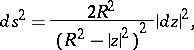which is invariant under conformal mappings and which defines the Lobachevskii geometry in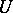.